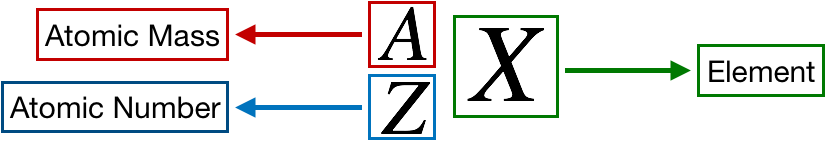# Problem: Explain the difference between Z (the atomic number) and A (the mass number).

###### FREE Expert Solution

We are asked to explain the difference between Z (the atomic number) and A (the mass number).###### Problem Details

Explain the difference between Z (the atomic number) and A (the mass number).